The Orca3D Hydrostatics & Stability module can be used to simulate a flotation experiment, where a hull is placed in the water and then weights are added at various locations to determine the equilibrium flotation condition. In addition to a report of the final sinkage, heel, and trim, Orca3D can either transform the model so that the Z=0 plane is the equilibrium flotation plane, or a plane can be inserted into the model that represents the equilibrium flotation plane.

The input requirements are:

• A hull model – the hull model can be made up of one or more surfaces, polysurfaces, meshes, and polymeshes. The model does not need to be closed (watertight). Small gaps between surfaces will usually result in small errors. If the deck is not modeled, it will not create a problem unless the resulting condition would cause the deck edge to become submerged. You must be certain that the outward normal direction is correct on all the surfaces or meshes. When using the dir command, all the arrows should be pointing into the water. If the outward normal is incorrect, that surface will deduct from displaced volume, rather than contributing to it.
• The initial flotation condition, including the vertical center of gravity (VCG) – The initial flotation condition is the condition that the vessel floats in before additional weights are added, and it can be specified as:
1. total weight and center of gravity (longitudinal, transverse, and vertical)
2. sinkage, trim, and heel, and VCG
3. total weight, trim or LCG, heel or TCG, and VCG
4. freeboards at two or three locations, and VCG (with only two locations, heel will be assumed to be 0)
5. a plane that you have drawn in Rhino to represent the flotation plane, and the VCG
• A list of additional weights to add, and the LCG, TCG, and VCG of each one
• The water density (salt water, fresh water, or user-defined). This can be set in the Orca3D Properties dialog (default is salt water).

The output will be:

• The Hydrostatics & Stability report, documenting the resulting equilibrium flotation plane by the total displacement, LCG, TCG, VCG, as well as sinkage, trim, and heel (see the Orca3D Help file to learn how these quantities are defined)
• The model transformed to the equilibrium flotation plane, or a plane inserted into the model to represent the equilibrium flotation plane along with text dots at the locations of the center of buoyancy, center of flotation, and center of gravity.

As the first step we will establish the initial flotation condition using the various methods described above. Following that we will add items at various locations and find the equilibrium flotation condition.

## Establishing the Initial Flotation Condition

### Example 1: The total weight and center of gravity is known from a weight study

In this example, the vessel’s total weight and center of gravity is known or estimated, perhaps as the result of a weight study.

1. Select the hull surfaces (again, be sure that the outward normals are correct, using the dir command. If they are incorrect, use the flip option to correct them). This can be a full model (port and starboard sides) or just one half of a symmetric hull.
2. Start the Hydrostatics & Stability command (in the Orca3D menu, select Stability -> Compute Hydrostatics and Stability)
3. Select the Weight, LCG, and TCG radio buttons. Enter the weight and CG information. If your hull is a half-model, check the “Mirror about Centerplane” box. Check the “Add Plane(s) Representing the Water Surface” box.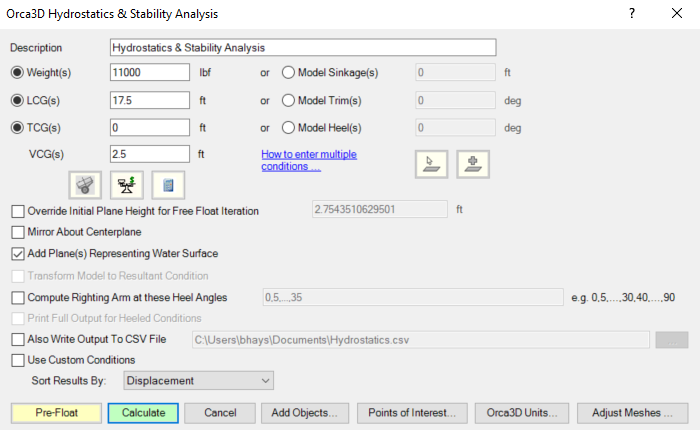4. Click Calculate; the Hydrostatics & Stability report will be created, and a cyan-colored plane will be inserted into the model representing the initial flotation condition. Verify that the flotation plane is as expected.

5. Now you are ready to move on to adding items to find the equilibrium flotation condition.### Example 2: The total weight and center of gravity is known using Orca3D’s Weight & Cost Tracking module

In this example, you have used Orca3D’s Weight & Cost model to assign materials from your material library, so that Orca3D can compute the weight and CG of each item in your model. This is then summed to get the total weight and CG.

1. If your Weight & Cost model represents the entire vessel (i.e., every item is included in the model), select the hull surfaces, and start the Hydrostatics & Stability command (in the Orca3D menu, select Stability -> Compute Hydrostatics and Stability).
2. Click on the “Get Weight/CG…” button. This will fill the total weight and CG from your Weight & Cost model into the fields for the Weight, LCG, TCG, and VCG. Note that if you have a half model, you will need to double the weight and adjust the TCG to 0.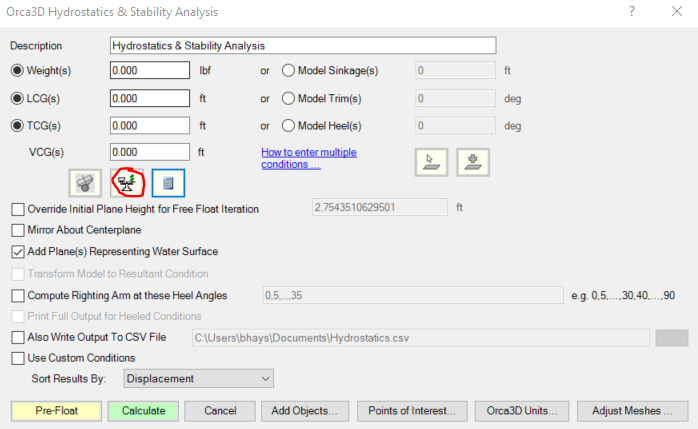3. If your hull is a half-model, check the “Mirror about Centerplane” box. Check the “Add Plane(s) Representing the Water Surface” box.

4. Click Calculate; the Hydrostatics & Stability report will be created, and a cyan-colored plane will be inserted into the model representing the initial flotation condition. Verify that the flotation plane is as expected.

5. Now you are ready to move on to adding items to find the equilibrium flotation condition.

6. If your Weight & Cost model does not represent the entire weight of the vessel, run a Weight & Cost report to get the modeled weight and CG.

7. Start the Hydrostatics & Stability command. Click on the Calculator icon: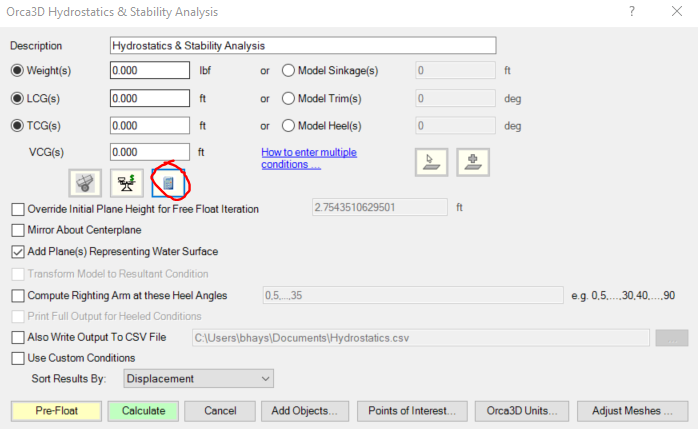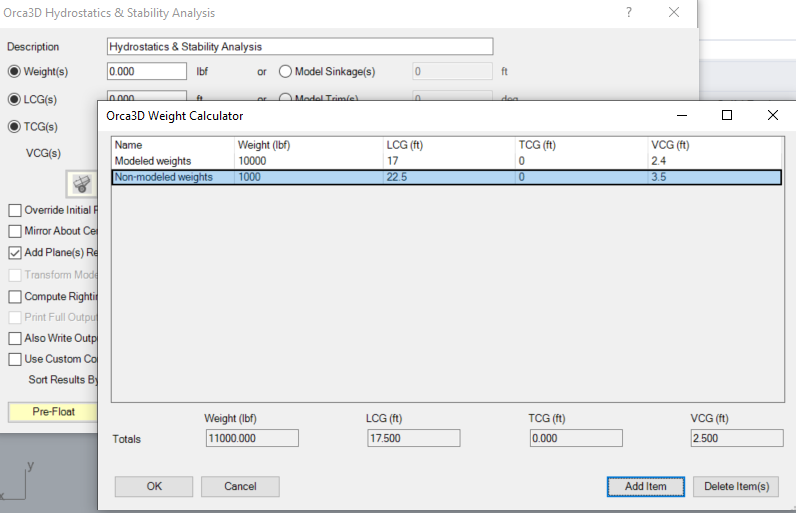8. The Orca3D Weight Calculator is essentially a simple spreadsheet. Click the Add Item button, and enter the Name, Weight, and CG that was shown in the Weight & Cost report (double it first, if you just have a half model).

9. Continue to add items to account for anything that was not included in the Weight & Cost model.

10. Click OK; the total Weight and CG will be entered into the Hydrostatics & Stability dialog.

11. If your hull is a half-model, check the “Mirror about Centerplane” box. Check the “Add Plane(s) Representing the Water Surface” box.

12. Click Calculate; the Hydrostatics & Stability report will be created, and a cyan-colored plane will be inserted into the model representing the initial flotation condition. Verify that the flotation plane is as expected.

13. Now you are ready to move on to adding items to find the equilibrium flotation condition.

### Example 3: The Sinkage, Trim, Heel, and VCG are known

In this example, the vessel’s Sinkage, Trim, Heel, and VCG are known. Frequently, this is because the vessel was modeled with the Z=0 plane at the design waterline, so the Sinkage, Trim, and Heel are 0.

1. Select the hull surfaces. This can be a full model (port and starboard sides) or just one half of a symmetric hull.
2. Start the Hydrostatics & Stability command (in the Orca3D menu, select Stability -> Compute Hydrostatics and Stability)
3. Select the Model Sinkage, Model Trim, and Model Heel radio buttons. Enter the values. If your hull is a half-model, check the “Mirror about Centerplane” box. Check the “Add Plane(s) Representing the Water Surface” box.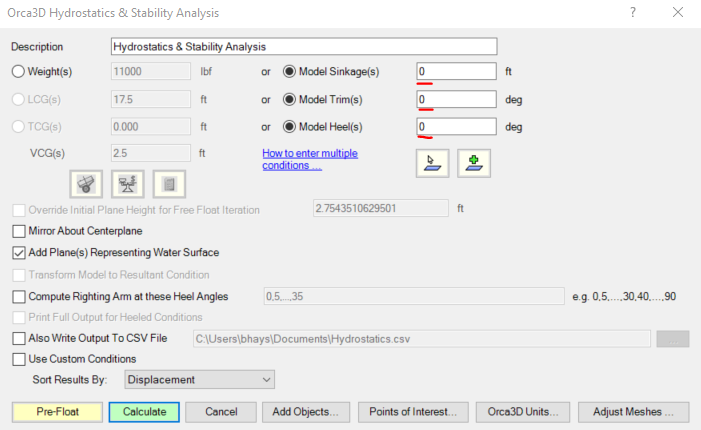4. Click Calculate; the Hydrostatics & Stability report will be created, and a cyan-colored plane will be inserted into the model representing the initial flotation condition. Verify that the flotation plane is as expected.

5. Now you are ready to move on to adding items to find the equilibrium flotation condition.

### Example 4: Freeboards are known from actual in-water measurements, and the VCG is known

In this example, the known information is freeboard measurements and the VCG. Remember that if the freeboards are known from actual measurements, it is important to set the water density (in Orca3D Properties) to match that of the water that the vessel was floating in when the freeboard measurements were taken. This will give an accurate weight, LCG, and TCG. If you want to analyze the effect of adding weights in a different water density (for example, your freeboards were taken in a fresh water tank, but you want the analysis in salt water), you can change the water density in the later step where you are finding the equilibrium flotation condition.

1. Select the hull surfaces. This can be a full model (port and starboard sides) or just one half of a symmetric hull.
2. Start the Hydrostatics & Stability command (in the Orca3D menu, select Stability -> Compute Hydrostatics and Stability)
3. Click on the “Create and select planar surface…” icon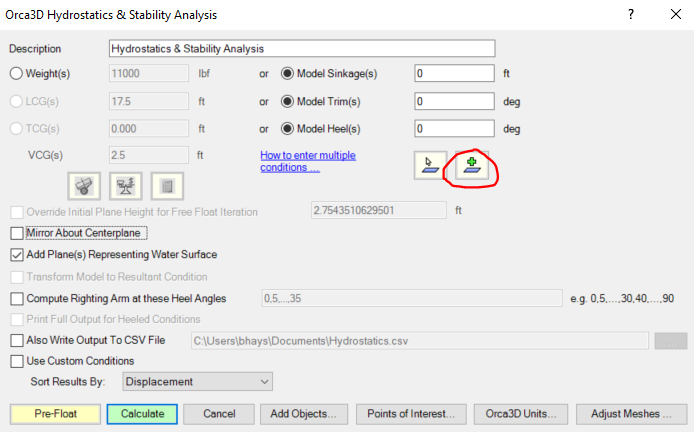4. Follow the prompts to select two or three points on the model where you have measured the freeboard and enter the distance to the water surface. If you finish the command after entering just two points, the vessel will be assumed to have 0 heel. If you enter three points, the heel will be computed, together with the sinkage and trim.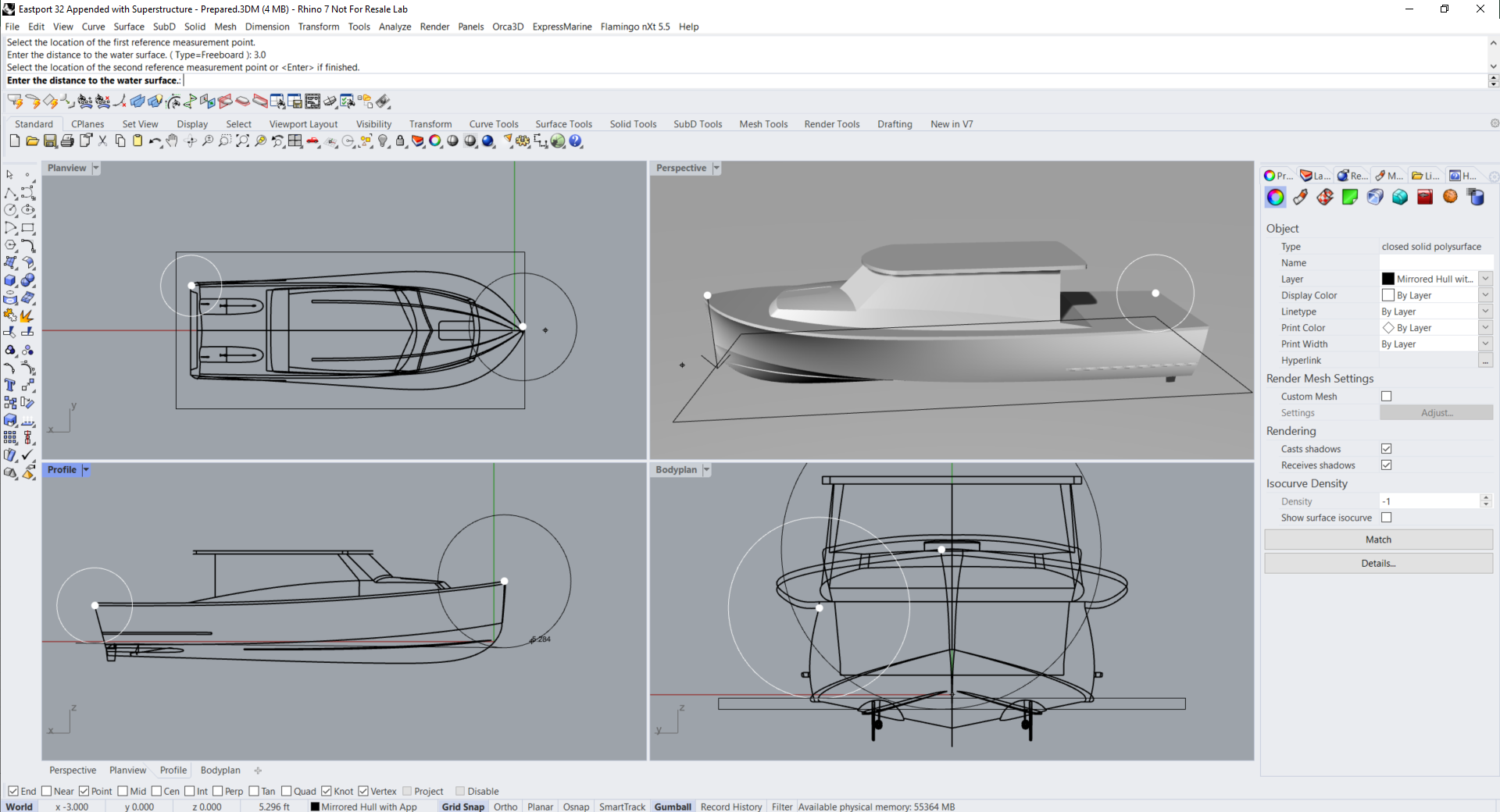5. When you finish entering the freeboard data, the computed Sinkage, Trim, and Heel will be entered into the fields.

6. If your hull is a half-model, check the “Mirror about Centerplane” box. Check the “Add Plane(s) Representing the Water Surface” box.

7. Click Calculate; the Hydrostatics & Stability report will be created, and a cyan-colored plane will be inserted into the model representing the initial flotation condition. Verify that the flotation plane is as expected.

8. Now you are ready to move on to adding items to find the equilibrium flotation condition.

### Example 5: The flotation plane and VCG are known

This is a variation of the previous example. Instead of entering freeboards to establish the flotation plane, in this example it is assumed that you have drawn a plane in the model that represents the initial flotation plane.

1. Select the hull surfaces. This can be a full model (port and starboard sides) or just one half of a symmetric hull.
2. Start the Hydrostatics & Stability command (in the Orca3D menu, select Stability -> Compute Hydrostatics and Stability)
3. Click on the “Select planar surface…” icon4. Click on the plane that represents the initial flotation condition.

5. When you select the plane, the computed Sinkage, Trim, and Heel will be entered into the fields.

6. If your hull is a half-model, check the “Mirror about Centerplane” box. Check the “Add Plane(s) Representing the Water Surface” box.

7. Click Calculate; the Hydrostatics & Stability report will be created, and a cyan-colored plane will be inserted into the model representing the initial flotation condition. Verify that the flotation plane is as expected.

8. Now you are ready to move on to adding items to find the equilibrium flotation condition.

## Adding Items to find the Equilibrium Flotation Condition

Now that the initial flotation condition has been established, we can move on to analyzing the effect of adding additional weights to the boat. As an example, we will add the following:

• 400 lb motorcycle (the example boat, courtesy of Eastport Yachts, conveniently features a transom gate)
• 150 lb inflatable boat with outboard engine (carried on the hard top)

Now that we know the initial flotation condition and VCG using one of the examples above, it is straightforward to analyze the effect of adding one or more additional items.

1. Select the hull surfaces. This can be a full model (port and starboard sides) or just one half of a symmetric hull.
2. Start the Hydrostatics & Stability command (in the Orca3D menu, select Stability -> Compute Hydrostatics and Stability)
3. If your hull is a half-model, check the “Mirror about Centerplane” box. Check the “Add Plane(s) Representing the Water Surface” box.
4. Select the Weight, LCG, and TCG radio buttons. Click the calculator icon:5. Enter the Weight and CG from the Initial Flotation Condition, as determined in the examples above.

6. Enter the list of additional weight items and their CG’s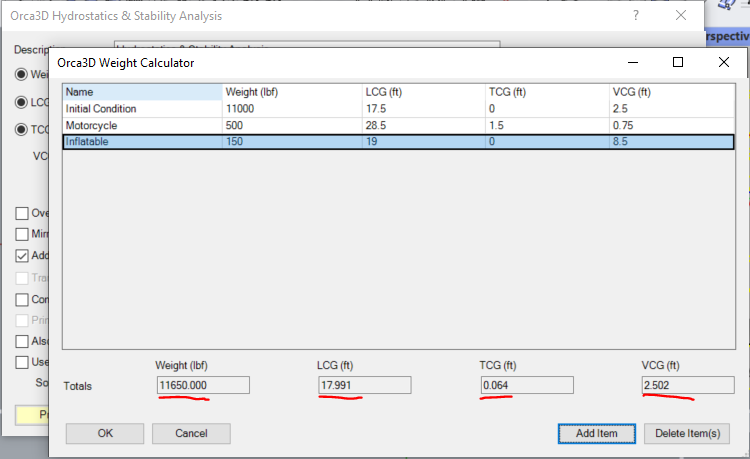7. Note that as you enter new items, the total weight and CG is updated at the bottom of the dialog.

8. Click OK; the total weight and CG will be entered into the appropriate fields.

9. Click OK; the Hydrostatics & Stability report will be created, showing the equilibrium flotation condition including Sinkage, Trim, and Heel. A cyan-colored plane will be displayed representing the water surface.

 By default, X is longitudinal, Y is transverse, and Z is the vertical coordinate. However, this is user-defined and it can be changed in the Orca3D Properties dialog. For the remainder of this document, the default orientation will be assumed.

 We will refer to the “hull surfaces” but this could include one or more surfaces, polysurfaces, meshes, or polysurfaces.